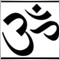# How to select by pos and compare 2 orders at a time? How to make a OrderSelect loop?745

How to select by pos and compare 2 orders at a time? How to make a OrderSelect loop?

Ex. I have i Total orders

for (int i=OrdersTotal()-1;i>=0; i--) {

I would like to loop comparison of (1st with 2nd) orders, (3rd with 4th), (5th with 6th), (7th with 8th), (9th with 10th) ....till  i, TotalOrder

(OrderSelect(0, SELECT_BY_POS))  // assuming the 0 is the first order (correct me if I am wrong)

and

(OrderSelect(1, SELECT_BY_POS))

then compare them

while still inside the total order loop

(OrderSelect(2, SELECT_BY_POS))   and

(OrderSelect(3, SELECT_BY_POS))

then compare

..... till i, TotalOrder

How to make a OrderSelect loop? The 0,1,2,3 above [in bold]

Basically I would like to compare (1st with 2nd) orders, (3rd with 4th), (5th with 6th), (7th with 8th), (9th with 10th) ....till  i, TotalOrder5299You need to decide what you want to compare.

You can only select one order at a time using orderselect().

Save the value you want to compare in a Variable.

Compare the Next value with the one you have saved.745

ubzen:

You need to decide what you want to compare.

You can only select one order at a time using orderselect().

Save the value you want to compare in a Variable.

Compare the Next value with the one you have saved.

Thanks
I know what I want to compare,

(1st with 2nd) orders, (3rd with 4th), (5th with 6th), (7th with 8th), (9th with 10th) ....till i, TotalOrder

Yes selecting one order at a time, but loop it. To select 1 and 2, compare, select 3 and 4 then compare .... until total order5299

It would look something like this.

```for(int a=OrdersTotal()-1;a>=0;a--){
if(1st_Compare_Whatever != 0){
2nd_Compare_Whatever=Value_You_Want_Compared;
if(1st_Compare_Whatever > 2nd_Compare_Whatever){
What_Ever_Count++;
1st_Compare_Whatever=0;
2nd_Compare_Whatever=0;
Continue;
}
}
1st_Compare_Whatever=Value_You_Want_Compared;
}
}```3200

johnnybegoode:

Thanks
I know what I want to compare,

(1st with 2nd) orders, (3rd with 4th), (5th with 6th), (7th with 8th), (9th with 10th) ....till i, TotalOrder

Yes selecting one order at a time, but loop it. To select 1 and 2, compare, select 3 and 4 then compare .... until total order

`for (int i = 0; i < OrdersTotal(); i += 2) `745

qjol:

thanks
nice that is a good idea, but how do I combine and compare them? Could I place selected order in a variable?

for (int i=OrdersTotal()-1;i>=0; i--) {

for (int i = 0; i < OrdersTotal(); i++){

(OrderSelect(i, SELECT_BY_POS)) = var A

for (int i = 0; i < OrdersTotal(); i += 2) {

(OrderSelect(i, SELECT_BY_POS)) = var B

ex.

if (OrderOpenPrice() of var A + OrderOpenPrice() of var B) > 5

{

then OrderClose both order 1 and 2

}745

ubzen:

It would look something like this.

then what about, 3 and 4,

5 and 6,

7 and 8 ...989

after selecting an order save the value: some_ticket = OrderTicket(); Then refer to your orders only by their ticket numbers. Use OrderSelect(some_ticket, SELECT_BY_TICKET); to quickly select an order before doing any operations on it. Trying to rely on which order is currently selected through some levels of nested loops and function calls is a sure way to get into trouble and make your head hurting while trying to understand what might be going on when something is wrong in your reasoning once the code gets more complicated.

Make it simple and robust by referring to orders only via their ticket number.3200

first let me understand what u what compare the OrderOpenPrice or the symbol or TP or what?3200

& B.T.W with this code:

for (int i=OrdersTotal()-1;i>=0; i--) {

u r selecting the last order745

7bit:
after selecting an order save the value of some_ticket = OrderTicket() in a variable. Then refer to orders only by their ticket number. use OrderSelect(some_ticket, SELECT_BY_TICKET); to quickly select an order before doing any operations on it. Trying to rely on which order is currently selected through some levels of nested loops and function calls is a sure way to get into trouble once the code gets more complicated.

thanks
nice that is a good idea, but how do I combine and compare them?

for (int i=OrdersTotal()-1;i>=0; i--) {

for (int i = 0; i < OrdersTotal(); i++){

(OrderSelect(i, SELECT_BY_TICKET)) = int ticket A

for (int i = 0; i < OrdersTotal(); i += 2) {

(OrderSelect(i, SELECT_BY_TICKET)) = int ticket B

ex.

if (OrderOpenPrice() of int ticket A + OrderOpenPrice() of int ticket B) > 5

{

then OrderClose both ticket A and ticket B

}